# How to set the Name of the RadioButton in C#?

In Windows Forms, RadioButton control is used to select a single option among the group of the options. For example, select your gender from the given list, so you will choose only one option among three options like Male or Female or Transgender. In Windows Forms, you are allowed to give a name to your RadioButton using the Name Property of the RadioButton control. You can set this property in two different ways:

1. Design-Time: It is the easiest way to set a name to RadioButton as shown in the below screenshots:

• Step 1: Create a windows form as shown in the below image:
Visual Studio -> File -> New -> Project -> WindowsFormApp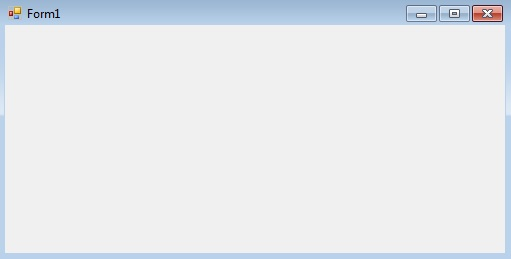• Step 2: Drag the RadioButton control from the ToolBox and drop it on the windows form. You are allowed to place a RadioButton control anywhere on the windows form according to your need.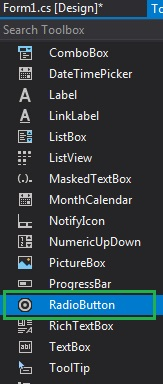• Step 3: After drag and drop you will go to the properties of the RadioButton control to set a name to RadioButton.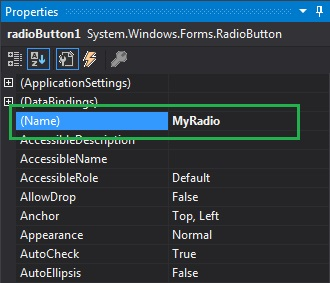Output: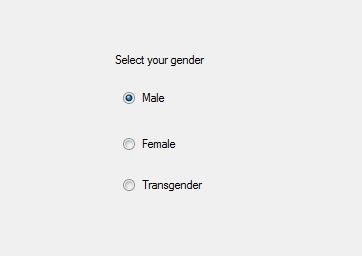2. Run-Time: It is a little bit trickier than the above method. In this method, you can set a name to RadioButton programmatically with the help of given syntax:

`public string Name { get; set; }`

The value of this property is of System.String type and this string represent the name of the RadioButton. The following steps show how to set a name of the RadioButton dynamically:

• Step 1: Create a radio button using the RadioButton() constructor is provided by the RadioButton class.
```// Creating radio button
```
• Step 2: After creating RadioButton, set the Name property of the RadioButton provided by the RadioButton class.
```// Setting the name of the radio button
```
```// Add this radio button to the form
```

Example:

 `using` `System; ` `using` `System.Collections.Generic; ` `using` `System.ComponentModel; ` `using` `System.Data; ` `using` `System.Drawing; ` `using` `System.Linq; ` `using` `System.Text; ` `using` `System.Threading.Tasks; ` `using` `System.Windows.Forms; ` ` `  `namespace` `WindowsFormsApp24 { ` ` `  `public` `partial` `class` `Form1 : Form { ` ` `  `    ``public` `Form1() ` `    ``{ ` `        ``InitializeComponent(); ` `    ``} ` ` `  `    ``private` `void` `RadioButton2_CheckedChanged(``object` `sender, ` `                                              ``EventArgs e) ` `    ``{ ` `    ``} ` ` `  `    ``private` `void` `Form1_Load(``object` `sender, EventArgs e) ` `    ``{ ` `        ``// Creating and setting label ` `        ``Label l = ``new` `Label(); ` `        ``l.AutoSize = ``true``; ` `        ``l.Location = ``new` `Point(176, 40); ` `        ``l.Text = ``"Select Post:"``; ` `        ``l.Font = ``new` `Font(``"Berlin Sans FB"``, 12); ` ` `  `        ``// Adding this label to the form ` `        ``this``.Controls.Add(l); ` ` `  `        ``// Creating and setting the  ` `        ``// properties of the RadioButton ` `        ``RadioButton r1 = ``new` `RadioButton(); ` `        ``r1.AutoSize = ``true``; ` `        ``r1.Text = ``"Intern"``; ` `        ``r1.Name = ``"My_Radio_1"``; ` `        ``r1.Location = ``new` `Point(286, 40); ` `        ``r1.Font = ``new` `Font(``"Berlin Sans FB"``, 12); ` ` `  `        ``// Adding this label to the form ` `        ``this``.Controls.Add(r1); ` ` `  `        ``// Creating and setting the ` `        ``// properties of the RadioButton ` `        ``RadioButton r2 = ``new` `RadioButton(); ` `        ``r2.AutoSize = ``true``; ` `        ``r2.Name = ``"My_Radio_2"``; ` `        ``r2.Text = ``"Team Leader"``; ` `        ``r2.Location = ``new` `Point(356, 40); ` `        ``r2.Font = ``new` `Font(``"Berlin Sans FB"``, 12); ` `        ``// Adding this label to the form ` `        ``this``.Controls.Add(r2); ` ` `  `        ``// Creating and setting the ` `        ``// properties of the RadioButton ` `        ``RadioButton r3 = ``new` `RadioButton(); ` `        ``r3.AutoSize = ``true``; ` `        ``r3.Name = ``"My_Radio_3"``; ` `        ``r3.Text = ``"Software Engineer"``; ` `        ``r3.Location = ``new` `Point(480, 40); ` `        ``r3.Font = ``new` `Font(``"Berlin Sans FB"``, 12); ` ` `  `        ``// Adding this label to the form ` `        ``this``.Controls.Add(r3); ` `    ``} ` `} ` `} `

Output: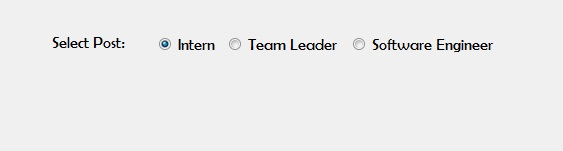My Personal Notes arrow_drop_upCheck out this Author's contributed articles.

If you like GeeksforGeeks and would like to contribute, you can also write an article using contribute.geeksforgeeks.org or mail your article to contribute@geeksforgeeks.org. See your article appearing on the GeeksforGeeks main page and help other Geeks.

Please Improve this article if you find anything incorrect by clicking on the "Improve Article" button below.

Article Tags :

Be the First to upvote.

Please write to us at contribute@geeksforgeeks.org to report any issue with the above content.